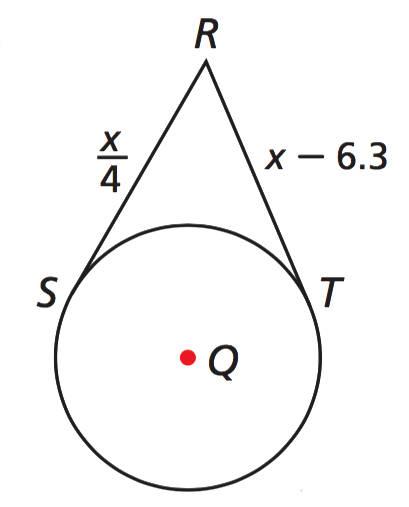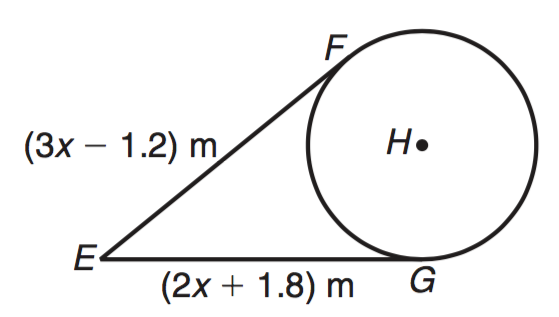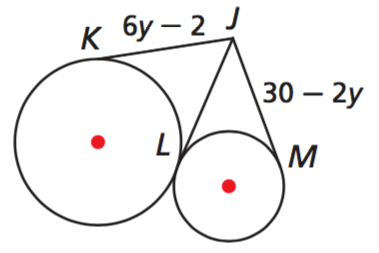Kindergarten
1st
2nd
3rd
4th
5th
6th
7th
8th
9th
10th
11th
12th
Higher Ed
Other
Subjects
ELA
Math
Science
Social Studies
Art
Computer Science
French
German
Music
Physical Education
Spanish
Other
Private Library
11-1 Lines that Intersect Circles
starstarstarstarstarstarstarstarstarstar
5 (1 rating)
by Jonathan Shaffer
| 30 Questions
Note from the author:
Introduction lesson to parts of circles including tangents, secants, chords, diameters, and radii.1
2 pts
Choose the options below that represent radii.

Line m
Line ℓ
2
1 pt
Which object below is a diameter?
Line ℓ
Line m
Segment FE
D
3
1 pt
Which object below represents a tangent line
Line ℓ
Line FE

Line m4
1 pt
Which object represents a diameter?

Line h

5
1 pt
Which object is a tangent line?
Line h
Line l
Line j
Line m
6
1 pt
Which object is a secent?

Line JM

Line JL7
1 pt
ED = 5.49 miles. Find EH. Round to the nearest whole mile.8
1 pt
Find the value of x.
9
1 pt
Find the measurement of RS.10
1 pt
Find the value of n.
11
1 pt
Find SR.12
1 pt
Find x.
13
1 pt
Find LJ.14
1 pt
Find y.
15
1 pt
Find SU.16
1 pt
Solve for x.
17
1 pt
Find EG.
Don't forget units.18
1 pt
Find the m∠Q.
Hint: QSPR is a quadrilateral. Review Theorem 11-1-1.19
1 pt
Find m∠P.
20
1 pt
Find m∠Q.21
1 pt
Find ST22
1 pt
Find DE23
1 pt
Find JL24
1 pt
Find the figure above, find QS.25
1 pt
The area of ⊙H is 100π cm². HF = 26cm. What is the individual measurement of EF and FG?
26
1 pt
What is the perimeter of quadilateral HEFG?27
1 pt
Find the value of x.
28
1 pt
Find JK
29
1 pt
Find y.
Hint: You'll have to factor to find the answer. There will only be one answer that gives a positive distance.
30
1 pt
Find IJ
Add to my formatives list